The Quilt Blocks ClipArt gallery offers 100 illustrations of geometric quilt-like patterns that can be used to demonstrate translation and rotation.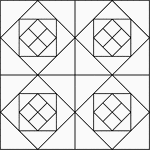Geometric Block Pattern 1

Geometric pattern for translation and rotation exercises.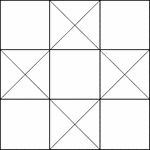Geometric Block Pattern 10

Geometric pattern for translation and rotation exercises.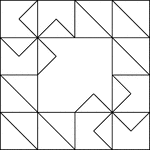Geometric Block Pattern 100

Geometric pattern for translation and rotation exercises.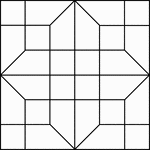Geometric Block Pattern 101

Geometric pattern for translation and rotation exercises.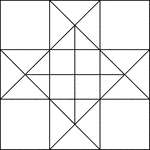Geometric Block Pattern 11

Geometric pattern for translation and rotation exercises.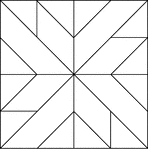Geometric Block Pattern 12

Geometric pattern for translation and rotation exercises.Geometric Block Pattern 13

Geometric pattern for translation and rotation exercises.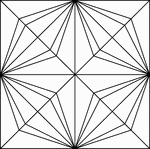Geometric Block Pattern 14

Geometric pattern for translation and rotation exercises.Geometric Block Pattern 15

Geometric pattern for translation and rotation exercises.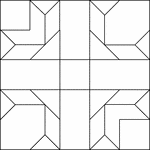Geometric Block Pattern 16

Geometric pattern for translation and rotation exercises.Geometric Block Pattern 17

Geometric pattern for translation and rotation exercises.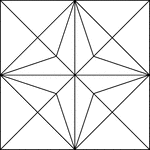Geometric Block Pattern 18

Geometric pattern for translation and rotation exercises.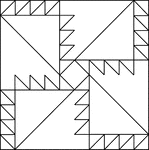Geometric Block Pattern 19

Geometric pattern for translation and rotation exercises.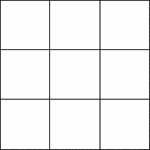Geometric Block Pattern 2

Geometric pattern for translation and rotation exercises.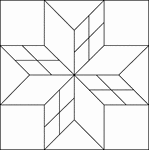Geometric Block Pattern 20

Geometric pattern for translation and rotation exercises.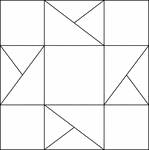Geometric Block Pattern 21

Geometric pattern for translation and rotation exercises.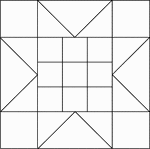Geometric Block Pattern 22

Geometric pattern for translation and rotation exercises.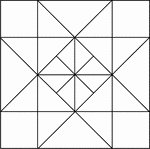Geometric Block Pattern 23

Geometric pattern for translation and rotation exercises.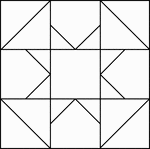Geometric Block Pattern 24

Geometric pattern for translation and rotation exercises.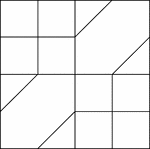Geometric Block Pattern 25

Geometric pattern for translation and rotation exercises.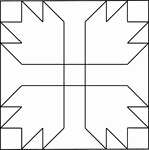Geometric Block Pattern 26

Geometric pattern for translation and rotation exercises.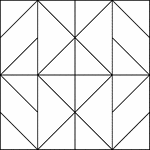Geometric Block Pattern 27

Geometric pattern for translation and rotation exercises.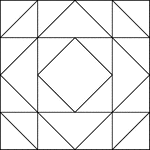Geometric Block Pattern 28

Geometric pattern for translation and rotation exercises.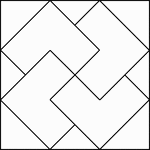Geometric Block Pattern 29

Geometric pattern for translation and rotation exercises.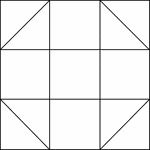Geometric Block Pattern 3

Geometric pattern for translation and rotation exercises.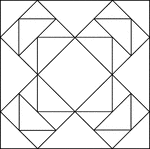Geometric Block Pattern 30

Geometric pattern for translation and rotation exercises.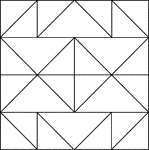Geometric Block Pattern 31

Geometric pattern for translation and rotation exercises.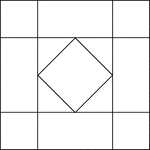Geometric Block Pattern 32

Geometric pattern for translation and rotation exercises.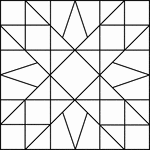Geometric Block Pattern 33

Geometric pattern for translation and rotation exercises.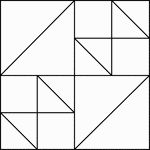Geometric Block Pattern 34

Geometric pattern for translation and rotation exercises.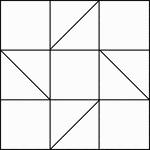Geometric Block Pattern 35

Geometric pattern for translation and rotation exercises.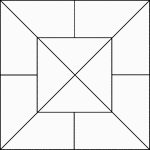Geometric Block Pattern 36

Geometric pattern for translation and rotation exercises.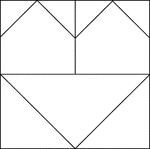Geometric Block Pattern 37

Geometric pattern for translation and rotation exercises.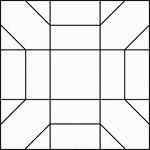Geometric Block Pattern 38

Geometric pattern for translation and rotation exercises.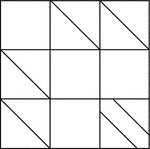Geometric Block Pattern 39

Geometric pattern for translation and rotation exercises.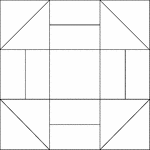Geometric Block Pattern 4

Geometric pattern for translation and rotation exercises.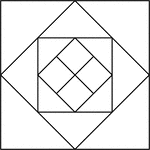Geometric Block Pattern 40

Geometric pattern for translation and rotation exercises.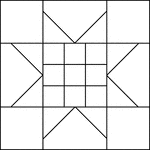Geometric Block Pattern 41

Geometric pattern for translation and rotation exercises.Geometric Block Pattern 42

Geometric pattern for translation and rotation exercises.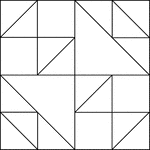Geometric Block Pattern 43

Geometric pattern for translation and rotation exercises.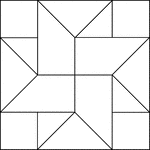Geometric Block Pattern 44

Geometric pattern for translation and rotation exercises.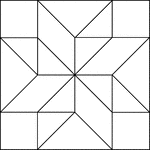Geometric Block Pattern 45

Geometric pattern for translation and rotation exercises.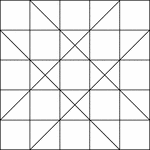Geometric Block Pattern 46

Geometric pattern for translation and rotation exercises.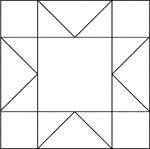Geometric Block Pattern 47

Geometric pattern for translation and rotation exercises.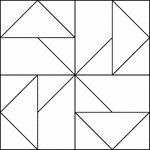Geometric Block Pattern 48

Geometric pattern for translation and rotation exercises.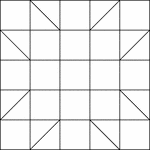Geometric Block Pattern 49

Geometric pattern for translation and rotation exercises.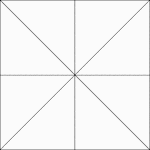Geometric Block Pattern 5

Geometric pattern for translation and rotation exercises.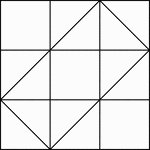Geometric Block Pattern 50

Geometric pattern for translation and rotation exercises.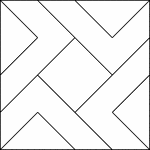Geometric Block Pattern 51

Geometric pattern for translation and rotation exercises.Geometric Block Pattern 52

Geometric pattern for translation and rotation exercises.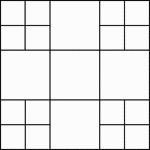Geometric Block Pattern 53

Geometric pattern for translation and rotation exercises.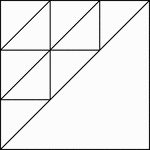Geometric Block Pattern 54

Geometric pattern for translation and rotation exercises.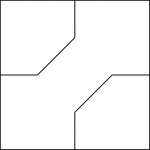Geometric Block Pattern 55

Geometric pattern for translation and rotation exercises.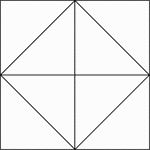Geometric Block Pattern 56

Geometric pattern for translation and rotation exercises.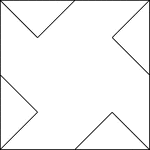Geometric Block Pattern 57

Geometric pattern for translation and rotation exercises.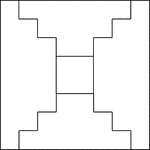Geometric Block Pattern 58

Geometric pattern for translation and rotation exercises.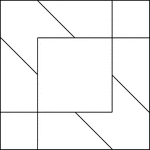Geometric Block Pattern 59

Geometric pattern for translation and rotation exercises.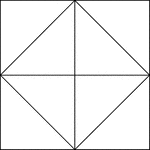Geometric Block Pattern 6

Geometric pattern for translation and rotation exercises.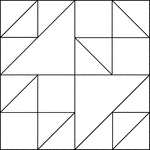Geometric Block Pattern 60

Geometric pattern for translation and rotation exercises.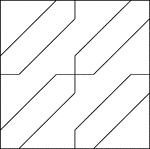Geometric Block Pattern 61

Geometric pattern for translation and rotation exercises.